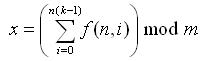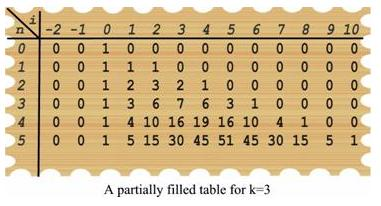Problems

# Hidden Truth in Recurrence

Time limit 1 second
Memory limit 128 MiB

You are given a recursive function, which has the following form:Now, you have to find: , where m = 10t.## Input data

Each line will contain three integers: k (0 < k < 10^19), n (0 < n < 10^19) and t (0 < t < 10). Input will be terminated by three zeros for the value of k, n and t. You must not process this case.

## Output data

For each line output the test case and the value of x. The output should be in the format shown in the sample output.

## Examples

Input example #1
1234 1234 4
2323 99999999999 8
4 99999 9
888 888 8
0 0 0

Output example #1
Case #1: 736
Case #2: 39087387
Case #3: 494777344
Case #4: 91255296